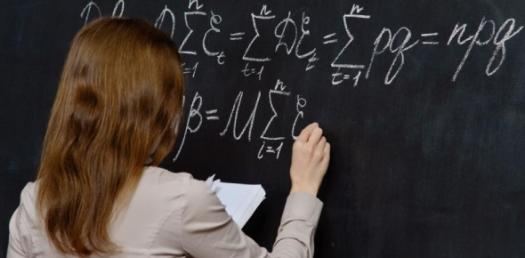# Math Trivia Quiz On Mensuration!

22 Questions | Total Attempts: 1813SettingsMensuration is a branch of math that is designed to help students understand how to carry out calculations that involve different geometrical shapes to get their area, volume, and parameters. Take up the quiz below and get to see just how skilled you are when it comes to geometry. Remember to share your results in the comment section.

• 1.
.The curved surface area of a right circular cylinder of radius 1cm and height 1cm is equal to  (1)                (2)                  (3)                   (4)
• A.

(2)

• B.

(3)

• C.

(1)

• D.

(4)

• 2.
The total surface area of a solid right circular cylinder whose radius is half its height h is equal to
• A.

3/2πh^2 sq.cm

• B.

3/2πhsq.cm

• C.

2/3πh^2 sq.cm

• D.

3/2πhsq.cm

• 3.
Base area of a right circular cylinder is 80   . If its height is 5cm,         Then the volume  is equal to
• A.

400 〖cm〗^3

• B.

16 〖cm〗^3

• C.

200 〖cm〗^3

• D.

400/3 〖cm〗^3

• 4.
If the total surface area a solid right circular cylinder is 200 π and its radius is 5 cm , then the sum of its height and radius is
• A.

20cm

• B.

25cm

• C.

30 cm

• D.

15 cm

• 5.
The curved surface area of a right circular cylinder whose radius is a units and height is b units,is equal to
• A.

2πab sq.cm

• B.

2π a^2 b sq.cm

• C.

2π sq.cm

• D.

2 sq.cm

• 6.
Radius and height of a right circular cone and that of a right circular cylinder are  respectively , equal. If the volume of the cylinder is 120  , then the volume of the cone  is equal to
• A.

40 〖cm〗^3

• B.

1200 〖cm〗^3

• C.

360 〖cm〗^3

• D.

90 〖cm〗^3

• 7.
If the diameter and height of a right circular cone are 12 cm and 8 cm  respectively , then the slant height is
• A.

10 cm

• B.

20 cm

• C.

30 cm

• D.

96 cm

• 8.
If the circumference at the base of a right circular cone and slant height are 120 π cm and 10 cm respectively, then the curved surface area of the cone is  equal to
• A.

600 π〖cm〗^2

• B.

1200 π〖cm〗^2

• C.

300 π〖cm〗^2

• D.

600〖cm〗^2

• 9.
If the volume and the base area of a right circular cone 48  π and 12 π   Respectively, then the height of the cone is
• A.

12 cm

• B.

10 cm

• C.

8 cm

• D.

6 cm

• 10.
If the height and the base area of a right circular cone are 5 cm and 48 sq.cm respectively, then the volume cone is
• A.

80〖cm〗^3

• B.

240〖cm〗^3

• C.

120 〖cm〗^3

• D.

480〖cm〗^3

• 11.
The ratio of the respective height and the respective radii of two cylinders are 1:2 and 2:1 respectively then the respective volumes are in the ratio
• A.

2:1

• B.

4:1

• C.

1:4

• D.

1:2

• 12.
If the radius of a sphere is 2 cm , then the curved surface area of the sphere is
• A.

16 π〖cm〗^2

• B.

12 π〖cm〗^2

• C.

16 〖cm〗^2

• D.

8 π〖cm〗^2

• 13.
The total surface area of a solid hemisphere of diameter 2 cm is equal to
• A.

3 π〖cm〗^2

• B.

4 π〖cm〗^2

• C.

12π〖cm〗^2

• D.

12〖cm〗^2

• 14.
If the volume of a  sphere is  π cu.cm , then its radius is
• A.

3/4 cm

• B.

4/3 cm

• C.

3/2 cm

• D.

2/3 cm

• 15.
The surface area of two spheres are in the ratio of 9:25. Then the volume are in the ratio
• A.

27:125

• B.

27:75

• C.

729:15625

• D.

81:625

• 16.
Total surface area of a solid hemisphere whose radius is a unit , is
• A.

3 πa^2 〖cm〗^2

• B.

3 πa〖cm〗^2

• C.

3a^2 〖cm〗^2

• D.

2 πa^2 〖cm〗^2

• 17.
If the surface area of a sphere is 100 π , then its radius is equal to
• A.

5 cm

• B.

10 cm

• C.

100 cm

• D.

25 cm

• 18.
If the surface area of a sphere is 36π , then the volume of the sphere is
• A.

36π〖cm〗^3

• B.

12π〖cm〗^3

• C.

72π 〖cm〗^3

• D.

108π〖cm〗^3

• 19.
If the total surface area of a solid hemisphere is 12π . Then its curved     Surface area is
• A.

8 π〖cm〗^2

• B.

36 π〖cm〗^2

• C.

24π 〖cm〗^2

• D.

6 π〖cm〗^2

• 20.
If the radius of the sphere is half of the radius of another sphere , then  their the respective volume are
• A.

1:8

• B.

2:1

• C.

1:2

• D.

8:1

• 21.
Curved surface area of a solid sphere is 24 . If the sphere divided into  Two hemispheres , then the total surface area of one of the hemisphere is
• A.

18 〖cm〗^2

• B.

16 〖cm〗^2

• C.

8〖cm〗^2

• D.

12〖cm〗^2

• 22.
Two right circular cones have equal radii . If the slant heights are in the ratio 4:3  , then their respective curved surface area are in the ratio
• A.

4:3

• B.

3:4

• C.

2:3

• D.

16:9

Related TopicsBack to top